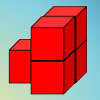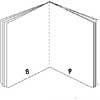Search by Topic

Resources tagged with Investigations similar to Midpoint Triangle:

Filter by: Content type:
Age range:
Challenge level:

There are 145 results

Broad Topics > Using, Applying and Reasoning about Mathematics > InvestigationsLittle Boxes

Age 7 to 11 Challenge Level:

How many different cuboids can you make when you use four CDs or DVDs? How about using five, then six?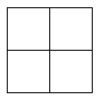Two Squared

Age 7 to 11 Challenge Level:

What happens to the area of a square if you double the length of the sides? Try the same thing with rectangles, diamonds and other shapes. How do the four smaller ones fit into the larger one?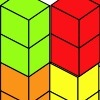Triple Cubes

Age 5 to 11 Challenge Level:

This challenge involves eight three-cube models made from interlocking cubes. Investigate different ways of putting the models together then compare your constructions.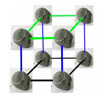More Pebbles

Age 7 to 11 Challenge Level:

Have a go at this 3D extension to the Pebbles problem.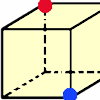Redblue

Age 7 to 11 Challenge Level:

Investigate the number of paths you can take from one vertex to another in these 3D shapes. Is it possible to take an odd number and an even number of paths to the same vertex?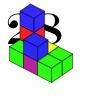28 and It's Upward and Onward

Age 7 to 11 Challenge Level:

Can you find ways of joining cubes together so that 28 faces are visible?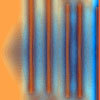Move a Match

Age 7 to 11 Challenge Level:

How can you arrange these 10 matches in four piles so that when you move one match from three of the piles into the fourth, you end up with the same arrangement?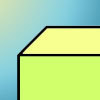Cuboid-in-a-box

Age 7 to 11 Challenge Level:

What is the smallest cuboid that you can put in this box so that you cannot fit another that's the same into it?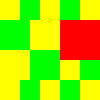Tiles on a Patio

Age 7 to 11 Challenge Level:

How many ways can you find of tiling the square patio, using square tiles of different sizes?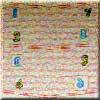Newspapers

Age 7 to 11 Challenge Level:

When newspaper pages get separated at home we have to try to sort them out and get things in the correct order. How many ways can we arrange these pages so that the numbering may be different?Calcunos

Age 7 to 11 Challenge Level:

If we had 16 light bars which digital numbers could we make? How will you know you've found them all?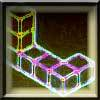Two on Five

Age 5 to 11 Challenge Level:

Take 5 cubes of one colour and 2 of another colour. How many different ways can you join them if the 5 must touch the table and the 2 must not touch the table?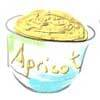Ice Cream

Age 7 to 11 Challenge Level:

You cannot choose a selection of ice cream flavours that includes totally what someone has already chosen. Have a go and find all the different ways in which seven children can have ice cream.Hexpentas

Age 5 to 11 Challenge Level:

How many different ways can you find of fitting five hexagons together? How will you know you have found all the ways?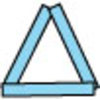Sticks and Triangles

Age 7 to 11 Challenge Level:

Using different numbers of sticks, how many different triangles are you able to make? Can you make any rules about the numbers of sticks that make the most triangles?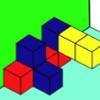Three Sets of Cubes, Two Surfaces

Age 7 to 11 Challenge Level:

How many models can you find which obey these rules?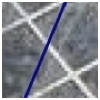Crossing the Town Square

Age 7 to 11 Challenge Level:

This tricky challenge asks you to find ways of going across rectangles, going through exactly ten squares.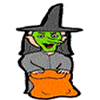Halloween Investigation

Age 7 to 11 Challenge Level:

Ana and Ross looked in a trunk in the attic. They found old cloaks and gowns, hats and masks. How many possible costumes could they make?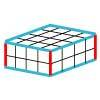Making Cuboids

Age 7 to 11 Challenge Level:

Let's say you can only use two different lengths - 2 units and 4 units. Using just these 2 lengths as the edges how many different cuboids can you make?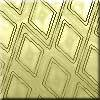Street Party

Age 7 to 11 Challenge Level:

The challenge here is to find as many routes as you can for a fence to go so that this town is divided up into two halves, each with 8 blocks.3 Rings

Age 7 to 11 Challenge Level:

If you have three circular objects, you could arrange them so that they are separate, touching, overlapping or inside each other. Can you investigate all the different possibilities?Room Doubling

Age 7 to 11 Challenge Level:

Investigate the different ways you could split up these rooms so that you have double the number.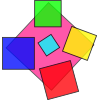Making Squares

Age 7 to 11

Investigate all the different squares you can make on this 5 by 5 grid by making your starting side go from the bottom left hand point. Can you find out the areas of all these squares?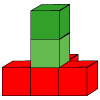Cubes Here and There

Age 7 to 11 Challenge Level:

How many shapes can you build from three red and two green cubes? Can you use what you've found out to predict the number for four red and two green?Tiling

Age 7 to 11 Challenge Level:

An investigation that gives you the opportunity to make and justify predictions.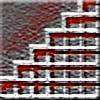Stairs

Age 5 to 11 Challenge Level:

This challenge is to design different step arrangements, which must go along a distance of 6 on the steps and must end up at 6 high.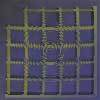My New Patio

Age 7 to 11 Challenge Level:

What is the smallest number of tiles needed to tile this patio? Can you investigate patios of different sizes?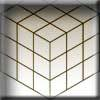Christmas Presents

Age 7 to 11 Challenge Level:

We need to wrap up this cube-shaped present, remembering that we can have no overlaps. What shapes can you find to use?Birthday Cake Candles

Age 7 to 11 Challenge Level:

This challenge involves calculating the number of candles needed on birthday cakes. It is an opportunity to explore numbers and discover new things.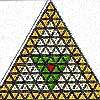The Great Tiling Count

Age 7 to 11 Challenge Level:

Compare the numbers of particular tiles in one or all of these three designs, inspired by the floor tiles of a church in Cambridge.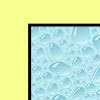All Wrapped Up

Age 7 to 11 Challenge Level:

What is the largest cuboid you can wrap in an A3 sheet of paper?Triangle Pin-down

Age 7 to 11 Challenge Level:

Use the interactivity to investigate what kinds of triangles can be drawn on peg boards with different numbers of pegs.Cubes

Age 7 to 11 Challenge Level:

How many faces can you see when you arrange these three cubes in different ways?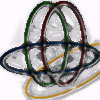Bean Bags for Bernard's Bag

Age 7 to 11 Challenge Level:

How could you put eight beanbags in the hoops so that there are four in the blue hoop, five in the red and six in the yellow? Can you find all the ways of doing this?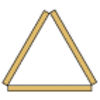Sticky Triangles

Age 7 to 11 Challenge Level:

Can you continue this pattern of triangles and begin to predict how many sticks are used for each new "layer"?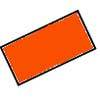Two by One

Age 7 to 11 Challenge Level:

An activity making various patterns with 2 x 1 rectangular tiles.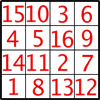Magic Constants

Age 7 to 11 Challenge Level:

In a Magic Square all the rows, columns and diagonals add to the 'Magic Constant'. How would you change the magic constant of this square?The Pied Piper of Hamelin

Age 7 to 11 Challenge Level:

This problem is based on the story of the Pied Piper of Hamelin. Investigate the different numbers of people and rats there could have been if you know how many legs there are altogether!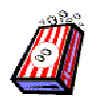Age 7 to 11 Challenge Level:

We went to the cinema and decided to buy some bags of popcorn so we asked about the prices. Investigate how much popcorn each bag holds so find out which we might have bought.It Figures

Age 7 to 11 Challenge Level:

Suppose we allow ourselves to use three numbers less than 10 and multiply them together. How many different products can you find? How do you know you've got them all?Age 7 to 11 Challenge Level:

I like to walk along the cracks of the paving stones, but not the outside edge of the path itself. How many different routes can you find for me to take?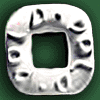Polo Square

Age 7 to 11 Challenge Level:

Arrange eight of the numbers between 1 and 9 in the Polo Square below so that each side adds to the same total.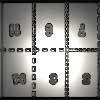Magazines

Age 7 to 11 Challenge Level:

Let's suppose that you are going to have a magazine which has 16 pages of A5 size. Can you find some different ways to make these pages? Investigate the pattern for each if you number the pages.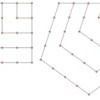Polygonals

Age 7 to 11 Challenge Level:

Polygonal numbers are those that are arranged in shapes as they enlarge. Explore the polygonal numbers drawn here.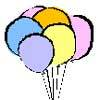Age 7 to 11 Challenge Level:

Lolla bought a balloon at the circus. She gave the clown six coins to pay for it. What could Lolla have paid for the balloon?Egyptian Rope

Age 7 to 11 Challenge Level:

The ancient Egyptians were said to make right-angled triangles using a rope with twelve equal sections divided by knots. What other triangles could you make if you had a rope like this?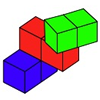Building with Rods

Age 7 to 11 Challenge Level:

In how many ways can you stack these rods, following the rules?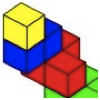Four Layers

Age 5 to 11 Challenge Level:

Can you create more models that follow these rules?